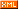# 简单，可复制

:: :: :: :: :: :::: ::431 随笔 :: 0 文章 :: 571 评论 :: 0 引用
java.util.regex是一个用正则表达式所订制的模式来对字符串进行匹配工作的类库包。它包括两个类：Pattern和Matcher Pattern 一个Pattern是一个正则表达式经编译后的表现模式。 Matcher 一个Matcher对象是一个状态机器，它依据Pattern对象做为匹配模式对字符串展开匹配检查。 首先一个Pattern实例订制了一个所用语法与PERL的类似的正则表达式经编译后的模式，然后一个Matcher实例在这个给定的Pattern实例的模式控制下进行字符串的匹配工作。

1        ((A)(B(C)))
2        (A)
3        (B(C))
4        (C)

java正则表达式通过java.util.regex包下的Pattern类与Matcher类实现(建议在阅读本文时,打开java API文档,当介绍到哪个方法时,查看java API中的方法说明,效果会更佳).
Pattern类用于创建一个正则表达式,也可以说创建一个匹配模式,它的构造方法是私有的,不可以直接创建,但可以通过Pattern.complie(String regex)简单工厂方法创建一个正则表达式,
Java代码示例:

Pattern p=Pattern.compile("\\w+");
p.pattern();//返回 \w+ 

pattern() 返回正则表达式的字符串形式,其实就是返回Pattern.complile(String regex)的regex参数

1.Pattern.split(CharSequence input)

Pattern有一个split(CharSequence input)方法,用于分隔字符串,并返回一个String[],我猜String.split(String regex)就是通过Pattern.split(CharSequence input)来实现的.
Java代码示例:

Pattern p=Pattern.compile("\\d+");
String[] str=p.split("我的QQ是:456456我的电话是:0532214我的邮箱是:aaa@aaa.com"); 

2.Pattern.matcher(String regex,CharSequence input)是一个静态方法,用于快速匹配字符串,该方法适合用于只匹配一次,且匹配全部字符串.

Java代码示例:

Pattern.matches("\\d+","2223");//返回true
Pattern.matches("\\d+","2223aa");//返回false,需要匹配到所有字符串才能返回true,这里aa不能匹配到
Pattern.matches("\\d+","22bb23");//返回false,需要匹配到所有字符串才能返回true,这里bb不能匹配到 

3.Pattern.matcher(CharSequence input)

Matcher类的构造方法也是私有的,不能随意创建,只能通过Pattern.matcher(CharSequence input)方法得到该类的实例.
Pattern类只能做一些简单的匹配操作,要想得到更强更便捷的正则匹配操作,那就需要将Pattern与Matcher一起合作.Matcher类提供了对正则表达式的分组支持,以及对正则表达式的多次匹配支持.
Java代码示例:

Pattern p=Pattern.compile("\\d+");
Matcher m=p.matcher("22bb23");
m.pattern();//返回p 也就是返回该Matcher对象是由哪个Pattern对象的创建的 

4.Matcher.matches()/ Matcher.lookingAt()/ Matcher.find()

Matcher类提供三个匹配操作方法,三个方法均返回boolean类型,当匹配到时返回true,没匹配到则返回false
matches()对整个字符串进行匹配,只有整个字符串都匹配了才返回true
Java代码示例:

Pattern p=Pattern.compile("\\d+");
Matcher m=p.matcher("22bb23");
m.matches();//返回false,因为bb不能被\d+匹配,导致整个字符串匹配未成功.
Matcher m2=p.matcher("2223");
m2.matches();//返回true,因为\d+匹配到了整个字符串

Pattern.compile(regex).matcher(input).matches()

lookingAt()对前面的字符串进行匹配,只有匹配到的字符串在最前面才返回true
Java代码示例:

Pattern p=Pattern.compile("\\d+");
Matcher m=p.matcher("22bb23");
m.lookingAt();//返回true,因为\d+匹配到了前面的22
Matcher m2=p.matcher("aa2223");
m2.lookingAt();//返回false,因为\d+不能匹配前面的aa 

find()对字符串进行匹配,匹配到的字符串可以在任何位置.
Java代码示例:

Pattern p=Pattern.compile("\\d+");
Matcher m=p.matcher("22bb23");
m.find();//返回true
Matcher m2=p.matcher("aa2223");
m2.find();//返回true
Matcher m3=p.matcher("aa2223bb");
m3.find();//返回true
Matcher m4=p.matcher("aabb");
m4.find();//返回false 

5.Mathcer.start()/ Matcher.end()/ Matcher.group()

start()返回匹配到的子字符串在字符串中的索引位置.
end()返回匹配到的子字符串的最后一个字符在字符串中的索引位置.
group()返回匹配到的子字符串
Java代码示例:

Pattern p=Pattern.compile("\\d+");
Matcher m=p.matcher("aaa2223bb");
m.find();//匹配2223
m.start();//返回3
m.end();//返回7,返回的是2223后的索引号
m.group();//返回2223

Mathcer m2=m.matcher("2223bb");
m.lookingAt();   //匹配2223
m.start();   //返回0,由于lookingAt()只能匹配前面的字符串,所以当使用lookingAt()匹配时,start()方法总是返回0
m.end();   //返回4
m.group();   //返回2223

Matcher m3=m.matcher("2223bb");
m.matches();   //匹配整个字符串
m.start();   //返回0,原因相信大家也清楚了
m.end();   //返回6,原因相信大家也清楚了,因为matches()需要匹配所有字符串
m.group();   //返回2223bb 

start(),end(),group()均有一个重载方法它们是start(int i),end(int i),group(int i)专用于分组操作,Mathcer类还有一个groupCount()用于返回有多少组.
Java代码示例:

Pattern p=Pattern.compile("([a-z]+)(\\d+)");
Matcher m=p.matcher("aaa2223bb");
m.find();   //匹配aaa2223
m.groupCount();   //返回2,因为有2组
m.start(1);   //返回0 返回第一组匹配到的子字符串在字符串中的索引号
m.start(2);   //返回3
m.end(1);   //返回3 返回第一组匹配到的子字符串的最后一个字符在字符串中的索引位置.
m.end(2);   //返回7
m.group(1);   //返回aaa,返回第一组匹配到的子字符串
m.group(2);   //返回2223,返回第二组匹配到的子字符串 

Java代码示例:

Pattern p=Pattern.compile("\\d+");
Matcher m=p.matcher("我的QQ是:456456 我的电话是:0532214 我的邮箱是:aaa123@aaa.com");
while(m.find()) {
System.out.println(m.group());
} 

456456
0532214
123 

while(m.find()) {
System.out.println(m.group());
System.out.print("start:"+m.start());
System.out.println(" end:"+m.end());
} 

456456
start:6 end:12
0532214
start:19 end:26
123
start:36 end:39 

posted on 2013-11-14 17:38 ggjucheng 阅读(...) 评论(...) 编辑 收藏